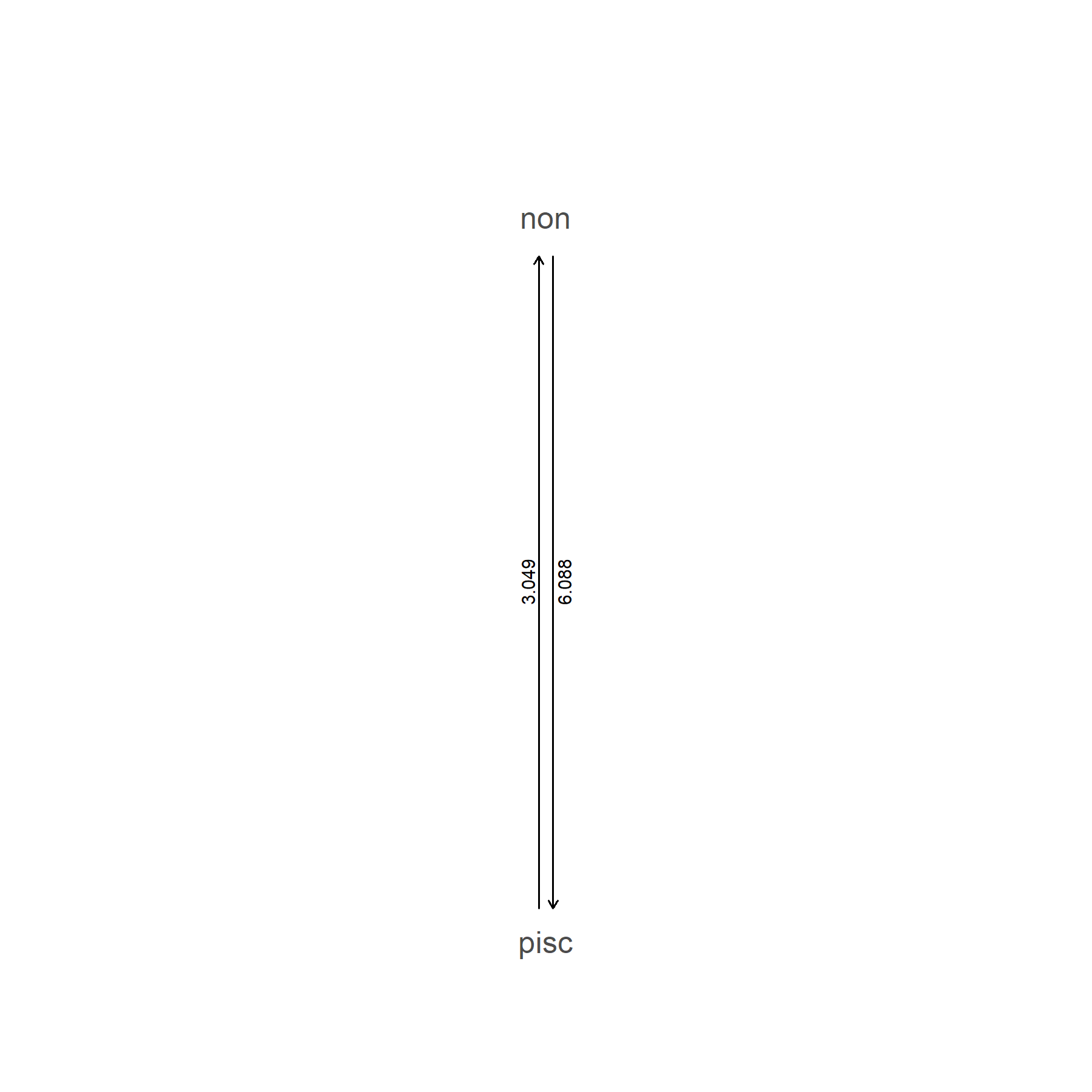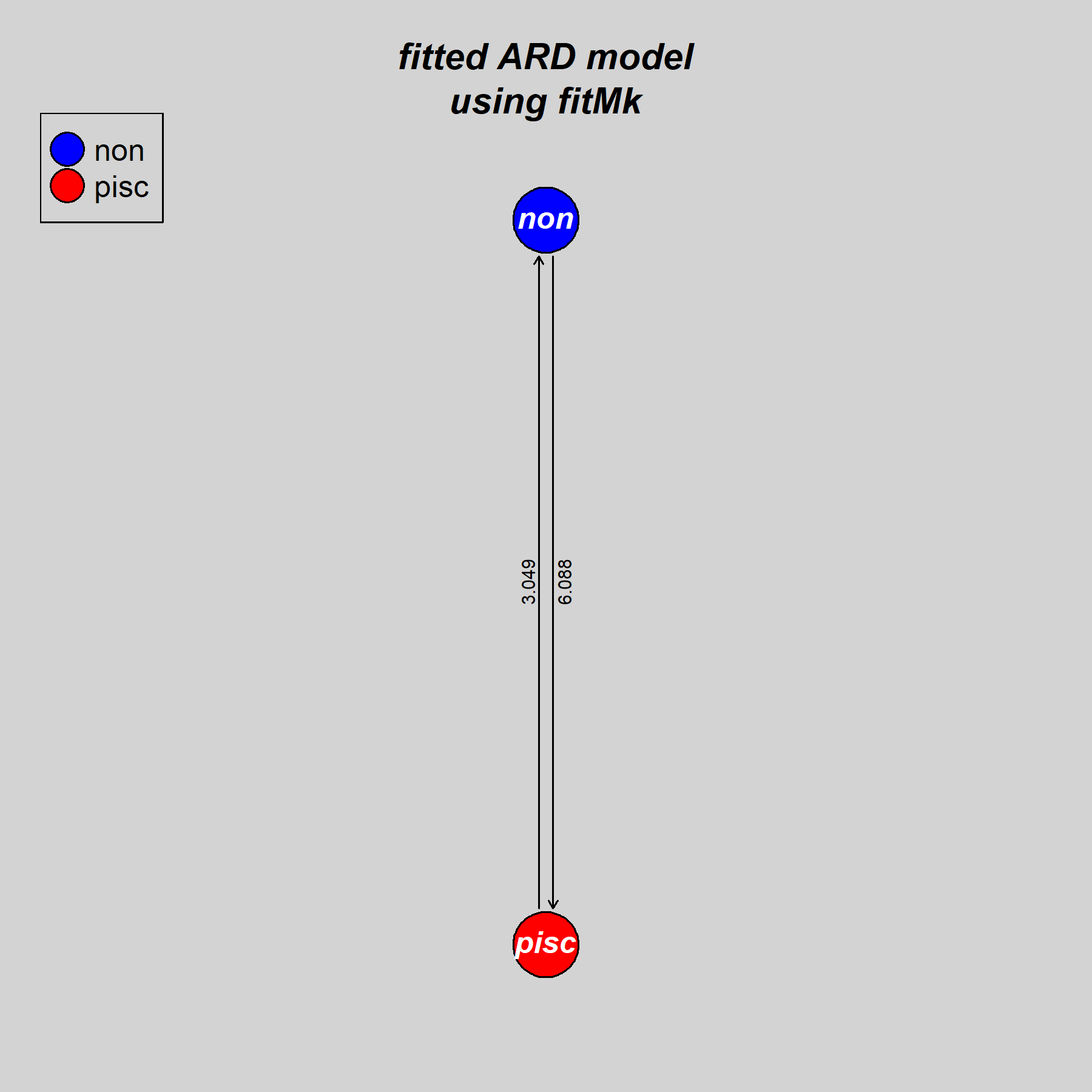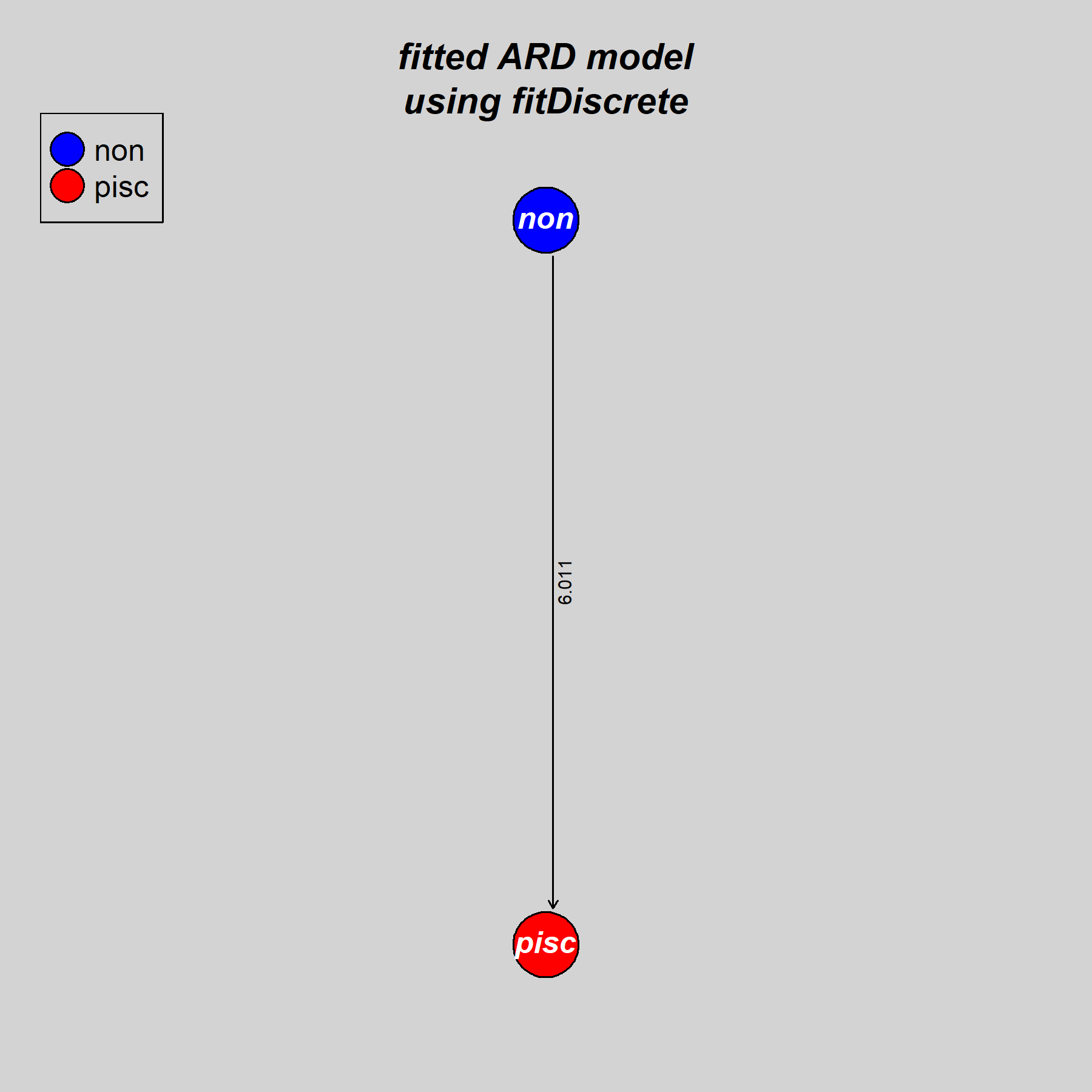## Wednesday, June 3, 2020

### Adding the Fitzjohn et al. root prior (π) to `phytools::fitMk`

Yesterday, when I posted about the new phytools object class `"Qmatrix"`, I happened to notice that `fitMk` and `fitDiscrete` produced (basically) the same fitted model for the evolution of ecomorph category in *Anolis lizards, in spite of the two different functions making really quite different assumptions about the root prior.

Just for fun, let's look at a different dataset in which the prior (normally denoted π) has a rather large effect. These data for feeding mode in Centrarchidae come from Revell & Collar (2009).

``````library(phytools)
data(sunfish.tree)
data(sunfish.data)
fmode<-setNames(sunfish.data\$feeding.mode,
rownames(sunfish.data))
``````

First, I'll fit & then plot the ARD model using `phytools::fitMk`:

``````fitARD.phytools<-fitMk(sunfish.tree,fmode,
model="ARD")
fitARD.phytools
``````
``````## Object of class "fitMk".
##
## Fitted (or set) value of Q:
##            non      pisc
## non  -6.087789  6.087789
## pisc  3.048905 -3.048905
##
## Fitted (or set) value of pi:
##  non pisc
##  0.5  0.5
## due to treating the root prior as (a) flat.
##
## Log-likelihood: -12.864937
##
## Optimization method used was "nlminb"
``````
``````plot(fitARD.phytools,show.zeros=FALSE)
``````Sorry, the plot is kind of boring for a binary trait. Let's jazz it up like we did yesterday:

``````par(bg="lightgrey")
coords<-plot(fitARD.phytools,show.zeros=FALSE)
library(plotrix)
nulo<-mapply(draw.circle,coords\$x,coords\$y,
text(coords\$x,coords\$y,coords\$states,col="white",
font=4)
legend("topleft",coords\$states,pch=21,pt.cex=2.5,
pt.bg=c("blue","red"))
title(main="fitted ARD model\nusing fitMk",font.main=4,
line=0)
``````Now let's do the same using `geiger::fitDiscrete`:

``````library(geiger)
fitARD.geiger<-fitDiscrete(sunfish.tree,fmode,
model="ARD")
fitARD.geiger
``````
``````## GEIGER-fitted comparative model of discrete data
##  fitted Q matrix:
##                    non          pisc
##     non  -6.011053e+00  6.011053e+00
##     pisc  1.400167e-14 -1.400167e-14
##
##  model summary:
##  log-likelihood = -12.295051
##  AIC = 28.590103
##  AICc = 29.070103
##  free parameters = 2
##
## Convergence diagnostics:
##  optimization iterations = 100
##  failed iterations = 0
##  number of iterations with same best fit = 32
##  frequency of best fit = 0.32
##
##  object summary:
##  'lik' -- likelihood function
##  'bnd' -- bounds for likelihood search
##  'res' -- optimization iteration summary
##  'opt' -- maximum likelihood parameter estimates
``````
``````par(bg="lightgrey")
coords<-plot(fitARD.geiger,show.zeros=FALSE)
library(plotrix)
nulo<-mapply(draw.circle,coords\$x,coords\$y,
text(coords\$x,coords\$y,coords\$states,col="white",
font=4)
legend("topleft",coords\$states,pch=21,pt.cex=2.5,
pt.bg=c("blue","red"))
title(main="fitted ARD model\nusing fitDiscrete",font.main=4,
line=0)
``````What the heck's going on? Believe it or not, it's all about the prior! By default, `phytools::fitMk` uses a flat prior whereas `geiger::fitDiscrete` uses a prior distribution cleverly suggested by Fitzjohn et al. (2009) in which π is basically treated as a nuisance parameter.

To prove this, I just added the Fitzjohn et al. prior to `fitMk`. Unfortunately, I did this today - so it comes too late to have been incorporated in the latest CRAN release of phytools. Nonetheless, it can already be obtained by updating phytools from GitHub using devtools.

Let's fit the Fitzjohn et al. prior model in `fitMk` and then extract the Q-matrix from that fit & our geiger fit:

``````fitARD.fitzjohn<-fitMk(sunfish.tree,fmode,
model="ARD",pi="fitzjohn")
fitARD.fitzjohn
``````
``````## Object of class "fitMk".
##
## Fitted (or set) value of Q:
##            non     pisc
## non  -6.011056 6.011056
## pisc  0.000000 0.000000
##
## Fitted (or set) value of pi:
##  non pisc
##    1    0
## due to treating the root prior as (a) nuisance.
##
## Log-likelihood: -12.295051
##
## Optimization method used was "nlminb"
``````
``````round(as.Qmatrix(fitARD.geiger),6)
``````
``````## Estimated Q matrix:
##            non     pisc
## non  -6.011053 6.011053
## pisc  0.000000 0.000000
``````

Cool.

OK then. Which model should we believe?